# Algebra 1 : How to find mode

## Example Questions

### Example Question #91 : How To Find Mode

What is the mode of the data set: 4 4 2 3 3 2 3 1 1 2 3 2 2 2 1 14 2Explanation:

To find the mode, count the number of times that each point appears in the data set:

2 is in the data set 7 times

3 is only in the data set 4 times

4 is only in the data set twice, and 1 appears 3 times.

### Example Question #92 : How To Find Mode

What is the mode of the following dataset?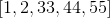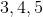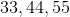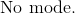Explanation:

The mode is the number or numbers with the highest frequency that occurs in the dataset.  Each number appears only once in this dataset.

Be careful not to include numbers that are not part of the dataset.

There is:### Example Question #91 : How To Find Mode

Find the mode of the following data set:

3, 9, 5, 4, 4, 10, 9, 2, 3, 1, 0, 9

12

4

9

0

10

9

Explanation:

The mode of a data set is simply the number that occurs most frequently.  In this case, the number 9 occurs most frequently, appearing 3 times.

Therefore, the mode of the given data set is 9.

### Example Question #92 : How To Find Mode

Find the mode: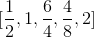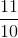Explanation:

The mode is the number or numbers that occur the most frequently in a dataset.

Simplify all the fractions in the dataset.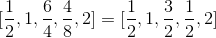We can then see thatoccurs twice, as every other number appears only once in the dataset.

The mode is:### Example Question #93 : How To Find Mode

Find the mode of the following set of numbers.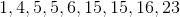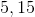Explanation:

To solve, simply find the most frequent number. Thus, you answer is bothandsince they both appear twice.

### Example Question #93 : How To Find Mode

Find the mode of the following set of numbers: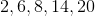None

None

Explanation:

The mode is the most frequent number. Since no number occurs more than another, the answer is none.

### Example Question #94 : How To Find Mode

This data set represents the quiz scores from a physics class.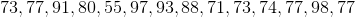What is the mode of this data set?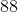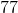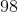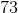Explanation:

This problem is asking us to solve for the data set's mode. The mode is merely the number that appears most often in a data set. One way to organize the information to easily see which number occurs most often is by ordering the numbers from least to greatest.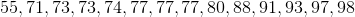Now we can easily identify that 77 occurs more often than any of the other numbers. Therefore the mode of the set is 77.

### Example Question #95 : How To Find Mode

A class of kindergarteners go to an apple orchard for a field trip. The students are allowed to pick apples. The following is a list of how many apples each student picked.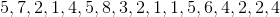What is the mode of this data set?Explanation:

This problem is asking us to solve for the mode for the data set consisting of how many apples each student picked. The mode is merely the number that appears most often in a data set. One way to organize the information to easily see which number occurs most often is by ordering the numbers from least to greatest.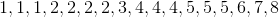Now we can easily identify that 2 occurs more often than any of the other numbers.

Therefore the mode of the set is 2.

### Example Question #96 : How To Find Mode

A group of students recorded data on the amount of rainfall that occurred in their town daily. They recorded the data in inches.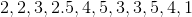What is the mode of their data set?Explanation:

This problem is asking us to solve for the data set's mode. The mode is merely the number that appears most often in a data set. One way to organize the information to easily see which number occurs most often is by ordering the numbers from least to greatest.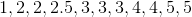Now we can easily identify that 3 occurs more often than any of the other numbers. Therefore the mode of the set is 3.

### Example Question #100 : How To Find Mode

Find the mode of the following dataset: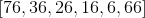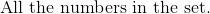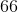Explanation:

The mode is the number or numbers with the highest occurrence in a dataset.

There are no numbers in this dataset that have an occurrence more than once.

Do not confuse mode with the amount of times a particular unit digit shows up.

The answer is:### All Algebra 1 Resources